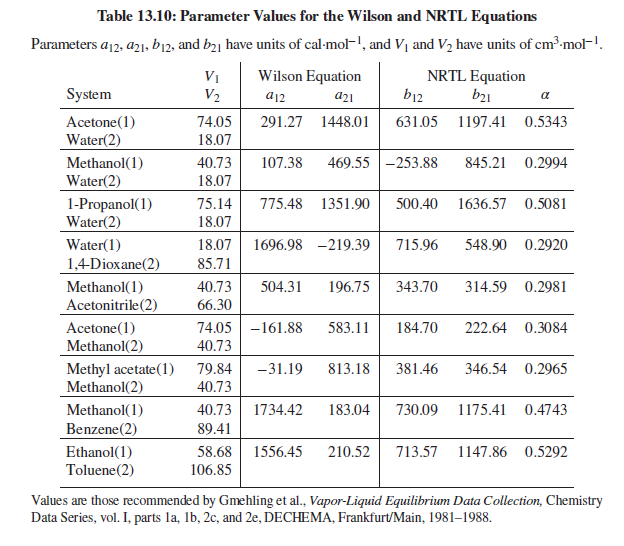×
Get Full Access to Introduction To Chemical Engineering Thermodynamics - 8 Edition - Chapter 13 - Problem 13.51
Get Full Access to Introduction To Chemical Engineering Thermodynamics - 8 Edition - Chapter 13 - Problem 13.51

×ISBN: 9781259696527 2049

## Solution for problem 13.51 Chapter 13

Introduction to Chemical Engineering Thermodynamics | 8th Edition

• Textbook Solutions
• 2901 Step-by-step solutions solved by professors and subject experts
• Get 24/7 help from StudySoup virtual teaching assistantsIntroduction to Chemical Engineering Thermodynamics | 8th Edition

4 5 1 279 Reviews
18
3
Problem 13.51

Problems 13.43 through 13.54 require parameter values for the Wilson or NRTL equation for liquid-phase activity coefficients. Table 13.10 gives parameter values for both equations. Antoine equations for vapor pressure are given in Table B.2, Appendix B.For the acetone(1)/methanol(2)/water(3) system, based on Eq. (13.19) and the Wilson equation, make the following calculations:

(a) BUBL P : $$t=65^{\circ} \mathrm{C}, x_{1}=0.3, x_{2}=0.4$$.

(b) DEW P : $$t=65^{\circ} C, y_{1}=0.3, y_{2}=0.4$$.

(c) P, T − flash : $$t=65^{\circ} \mathrm{C}, P=\frac{1}{2}\left(P_{\text {bubble }}+P_{\text {dew }}\right), z_{1}=0.3, z_{2}=0.4$$.

Text Transcription:

t=65^circ C, x_1=0.3, x_2=0.4

t=65^circ C, y_1=0.3, y_2=0.4

t=65^circ C, P=1/2(P_bubble+P_dew), z_1=0.3, z_2=0.4

Step-by-Step Solution:
Step 1 of 3

ENG 244 1/23/18 Take Home Quiz Prep Reading Due Today: Pat Kuris, “The Moko Returns: More than a Tattoo” and Te Awekotuku Mau Moko: The World of Maori Tattoo Ku’ualoha ho’omanawanui, “Tatz”  FIRST IMPRESSIONS o Written in Hawaiian Creole English (HCE) o Imagery, juxtaposition, writing w/ images o Kakau- tattoo  Noun and a verb o Araul vs Oral and Vision- reader must use the senses to understand the work o “no moa pepa” (21)- Body becomes the text o Genealogy involves geography, time, and family  Tattoos mark you and tell the story of both family and community o Cosmogonic Connections  “experience” (15) is “tapped into dea skill like genealogy” (5-7)  “genealogy, dead ancestors, ancestors, ancestry, tutu  ancestahs, like kalo o Haloa  First human, but was still born  His body was buried and Taro grew from his burial site  Humanity and the land have a brother/sister and give/take relationship o Parts of the body in poem- skin, blood, arm, thigh, back o One’s body belongs to the community o Investigation of permanence of Tatz versus Paper o Repetition of “Like” significant, shows metaphor o Enjambment- Free and uninterrupted carry over of syntax from one line to another Cross of Soot  Main points, influe

Step 2 of 3

Step 3 of 3

## Discover and learn what students are asking

Calculus: Early Transcendental Functions : Hyperbolic Functions
?In Exercises 7-14, verify the identity. $$\cosh ^{2} x=\frac{1+\cosh 2 x}{2}$$

Statistics: Informed Decisions Using Data : Bias in Sampling
?Discuss the benefits of having trained interviewers.

Unlock Textbook Solution